Courses

# Seepage in Soils Civil Engineering (CE) Notes | EduRev

## Civil Engineering (CE) : Seepage in Soils Civil Engineering (CE) Notes | EduRev

The document Seepage in Soils Civil Engineering (CE) Notes | EduRev is a part of the Civil Engineering (CE) Course Soil Mechanics.
All you need of Civil Engineering (CE) at this link: Civil Engineering (CE)

Seepage in Soils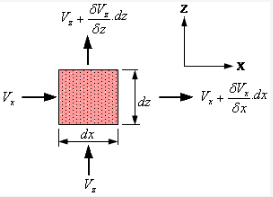A rectangular soil element is shown with dimensions dx and dz in the plane, and thickness dy perpendicuar to this plane. Consider planar flow into the rectangular soil element.

In the x-direction, the net amount of the water entering and leaving the element is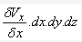Similarly in the z-direction, the difference between the water inflow and outflow is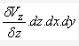For a two-dimensional steady flow of pore water, any imbalance in flows into and out of an element in the z-direction must be compensated by a corresponding opposite imbalance in the x-direction. Combining the above, and dividing by dx.dy.dz , the continuity equation is expressed as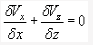From Darcy's law, ,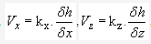, where h is the head causing flow.

when the continuity equation is combined with Darcy's law, the equation for flow is expressed as: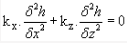For an isotropic material in which the permeability is the same in all directions (i.e. k x= k z), the flow equation is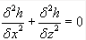This is the Laplace equation governing two-dimensional steady state flow. It can be solved graphically, analytically, numerically, or analogically.

For the more general situation involving three-dimensional steady flow, Laplace equation becomes: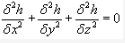One-dimensional Flow

For this, the Laplace Equation is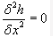Integrating twice, a general solution is obtained.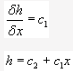The values of constants can be determined from the specific boundary conditions.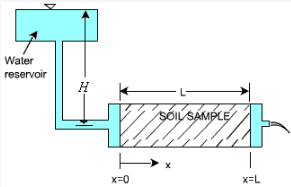As shown, at x = 0, h = H , and at = L, = 0

Substituting and solving,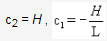The specific solution for flow in the above permeameter is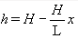which states that head is dissipated in a linearly uniform manner over the entire length of the permeameter.

Flow Nets
Graphical form of solutions to Laplace equation for two-dimensional seepage can be presented as flow nets. Two orthogonal sets of curves form a flow net:

• Equipotential lines connecting points of equal total head h
• Flow lines indicating the direction of seepage down a hydraulic gradient

Two flow lines can never meet and similarly, two equipotential lines can never meet. The space between two adjacent flow lines is known as a flow channel, and the figure formed on the flownet between any two adjacent flow lines and two adjacent equipotential lines is referred to as a field. Seepage through an embankment dam is shown.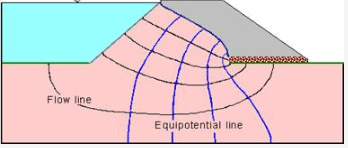Calculation of flow in a channel
If standpipe piezometers were inserted into the ground with their tips on a single equipotential line, then the water would rise to the same level in each standpipe. The pore pressures would be different because of their different elevations.There can be no flow along an equipotential line as there is no hydraulic gradient.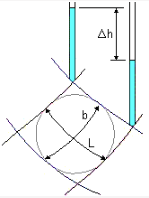Consider a field of length L within a flow channel. There is a fall of total head Dh. The average hydraulic gradient is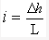As the flow lines are b apart and considering unit length perpendicuar to field, the flow rate is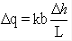There is an advantage in sketching flow nets in the form of curvilinear 'squares' so that a circle can be insrcibed within each four-sided figure bounded by two equipotential lines and two flow lines.

In such a square, b = L , and the flow rate is obtained as Dq = k.Dh

Thus the flow rate through such a flow channel is the permeability k multiplied by the uniform interval Dh between adjacent equipotential lines.

Calculation of total flow
For a complete problem, the flow net can be drawn with the overall head drop h divided into Nd so that Dh = h / Nd
If Nf is the no. of flow channels, then the total flow rate is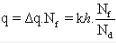Offer running on EduRev: Apply code STAYHOME200 to get INR 200 off on our premium plan EduRev Infinity!

## Soil Mechanics

31 videos|48 docs|42 tests

,

,

,

,

,

,

,

,

,

,

,

,

,

,

,

,

,

,

,

,

,

;